Range

In the cells that follow we see Range can be used in a lot of cases where
Table is often used.  One difference  is that Range will normally make a list
in about half the time Table would need to make the same list.

Range doesn't  have to count over integers.  In the next line Range starts at
0 and counts in steps of 1/20  up to the last value less than (π/3).
Notice (1<π/3), but (21/20>π/3), sot the list stops at 1.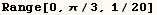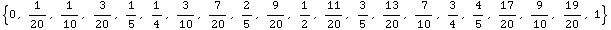The next example is similar to the previous example.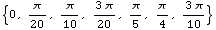In the same line where Range makes a list, a listable function such as Times
or Power can be applied to the list as in the next line. The same list can be
made using Table, but Range is almost always faster.  If the function being
applied to each element of the list isn't listable, you can map it over the
list, and it will work about as fast as if it was listable.  In the two
following examples Power is applied to the list returned by Range.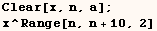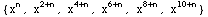The next line give an interesting example.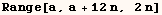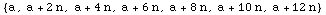In the next line Range is used to make a series expansion for Cos[x].   Timing experiments show that this is much faster than using Series, but the  method below is fairly cryptic.  Also creating the series using Range  requires knowledge of the general form of each term, while Series doesn't  need this information.  The fact that Series must determine the coefficients  for each term is probably the reason why it takes much longer.  The #&  notation is explained in the discussion of Function.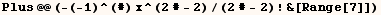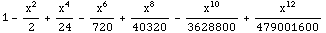Created by Mathematica  (May 16, 2004)

Back to Ted’s Tricks index page